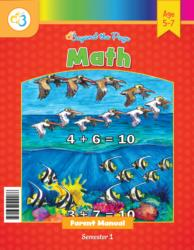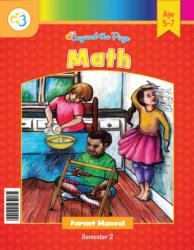# Common Core Alignment

CCSS.Math.Content.1.OA.6 - Add and subtract within 20, demonstrating fluency for addition and subtraction within 10. Use strategies such as counting on; making ten (e.g., 8 + 6 = 8 + 2 + 4 = 10 + 4 = 14); decomposing a number leading to a ten (e.g., 13 – 4 = 13 – 3 – 1 = 10 – 1 = 9); using the relationship between addition and subtraction (e.g., knowing that 8 + 4 = 12, one knows 12 – 8 = 4); and creating equivalent but easier or known sums (e.g., adding 6 + 7 by creating the known equivalent 6 + 6 + 1 = 12 + 1 = 13).

## 5: MathUnit 3: Addition and Subtraction to 10
Lesson 3: Getting on Board with Addition
Lesson 4: More Practice with Addition
Lesson 5: Finding DifferencesUnit 6: Time
Lesson 1: What Is Time?
Lesson 2: So Many Hours in a Day!
Lesson 3: Half Hours
Lesson 4: More With Half Hours
Lesson 5: More Work With TimeUnit 7: Place Value
Lesson 5: Practice Time!